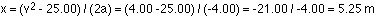## Introduction to Physics

9-3-99

Sections 1.1 - 1.8 and Appendix A

If you were taking a trip to Greece, you'd get the most out of your trip if you learned some Greek before going. Knowing a little of the language would help you somewhat; being fluent in the language would help you immensely. The same holds true for physics.This introduction to physics is simply a review of the language of physics. If you're unfamiliar with some of these concepts, spending the time to become fluent will help you immensely in the course. Practice makes perfect!

### MKS units and the metric system

MKS (meter-kilogram-second) units are part of the metric system, based on powers of ten to keep things simple. The more common prefixes used in the metric system, and the powers of ten associated with them, are given in the table below. You should know all of these off by heart.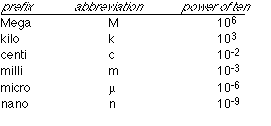### Unit analysis and conversion

You will often need to convert a value from one unit to another, converting from centimeters to meters, for example. Be extra careful if you have units which are squared, cubed, or have some other exponent. If you have a cube which is 10 cm on each side, then the volume is simply the length x the width x the height:

V = 10 cm x 10 cm x 10 cm = 1000 cm3.

In m3, the volume is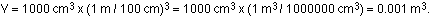Consider a slightly more complicated example, with two steps rather than one. When he set the 200-meter world record, 19.32 seconds, Michael Johnson ran at an average speed of 200 m / 19.32 s = 10.35 m/s. If you want to know how fast this is in miles/hour, the conversion would be carried out like this:

10.35 m/s x (1 mile / 1609 m) x (3600 s / 1 hour) = 23.2 miles / hour.

All you do to convert is multiply the original value by x/y, where x and y are the same thing, expressed in different units. Something else to keep in mind is that when values are multiplied or divided, they can have different units. When you add or subtract values, however, the values must have the same units.

### Significant figures

When you punch in 200/19.32 in your calculator, your calculator gives you the number 10.35196687. You have to round this off (10.35 is a good choice), because most of the figures the calculator gives you are not significant, meaning, essentially, that they're meaningless. The calculator assumes that what you type in is accurate to about 12 figures; typically, your numbers are accurate to 2 or 3 or 4 figures.

When you're combining numbers, the general rule of thumb is that you should round off your answers to the same number of significant figures as there are in the number with the smallest number of significant figures.

Another general rule of thumb : if you have to calculate intermediate values before you get to a final value, keep one or two extra significant figures for the intermediate values, just so you won't introduce any inaccuracies in the final answer by rounding off too soon.

### Trigonometry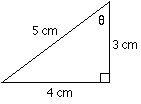Basic trigonometry is usually introduced by looking at a right-angled triangle. Let's use a triangle with sides of length 3 cm, 4 cm, and 5 cm. This satisfies the Pythagorean theorem, which states that for a right-angled triangle with sides a and b, and with a hypotenuse c (the side opposite the 90ˇ angle), the square of the hypotenuse equals the sum of the squares of the other two sides.Let's say we'd like to figure out the angle between the 3 cm side and the 5 cm side. We'll call this angle by the Greek letter theta. For a right-angled triangle, we can use the following relationships: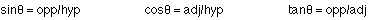where hyp stands for hypotenuse, opp stands for the side opposite to, and adj stands for the side adjacent to(the adjacent side that's not the hypotenuse). Note that the units cancel out, so these values have no units.

If we wanted to find the third angle in the triangle, we could use trigonometry, or geometry, remembering that the three angles in a triangle must add up to 180ˇ.

### The Sine law and the Cosine law

The sine relationship used above for the right-angled triangle, as well as the Pythagorean theorem, are simply special cases of the sine law and the cosine law, respectively. Most triangles do not have 90ˇ angles in them, but there are still ways to relate sides and angles. In any triangle the sine and cosine laws are as follows: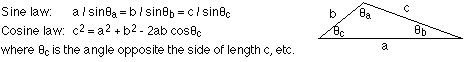Be extra careful with the sine law. If the angle is greater than 90ˇ, your calculator will give you 180 - the angle, because your calculator gives you an angle less than 90ˇ. To find the angle, subtract what your calculator gives you from 180ˇ.

### Algebra

Algebra involves the manipulation of equations to solve for unknown variables. PY105 involves a great deal of problem solving, requiring a lot of reasonably straightforward algebra. An example will help illustrate the sort of manipulations you'll be expected to carry out. Let's say you have an equation that states: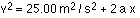and you're given a = -2.00 m/s2 and v = 2.00 m/s. You don't know x, so you have to rearrange the equation to solve for x, which means getting x by itself on the left side. Note that the equation involves numbers, variables, and units...it would be easy to confuse a unit with a variable, but usually it's fairly obvious what is what. In this case, v, a, and x are variables, while m and s are units (meters and seconds).

First of all, let's analyze the units. The left hand side has units of m2/s2, and the first term on the right does also. For the equation to make sense, the second term on the right must have the same units, which means x must have units of m. x is a length, in other words.

You don't have to do this in one step, but you should be able to re-arrange the equation above to solve for x: